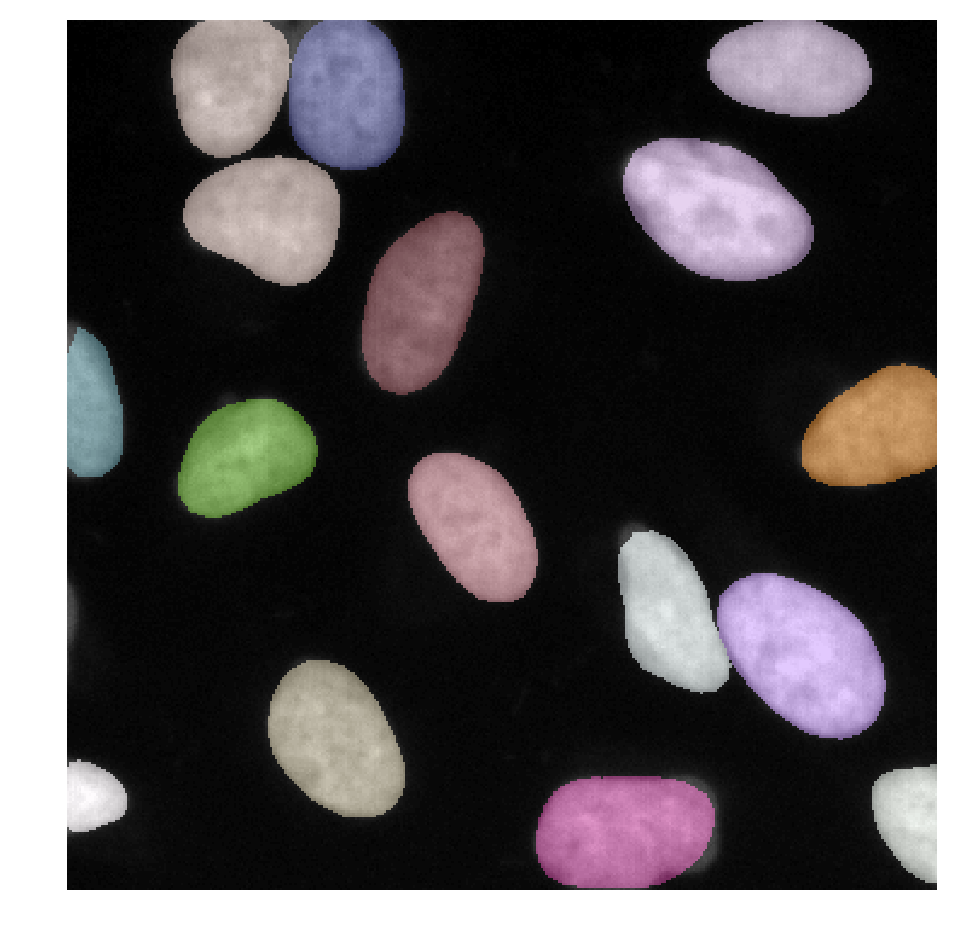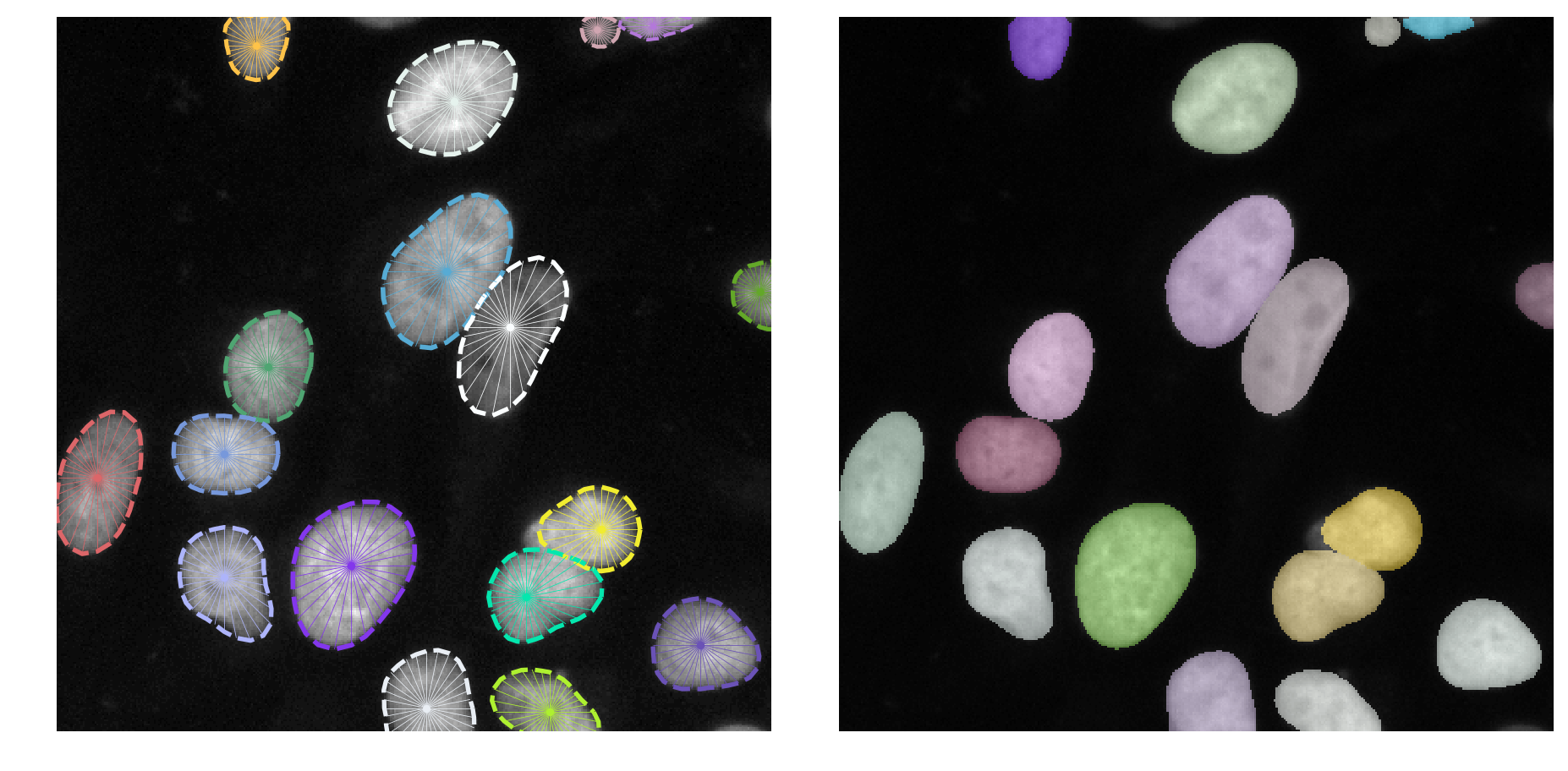In case of problems or questions, please first check the list of Frequently Asked Questions (FAQ).

Please shutdown all other training/prediction notebooks before running this notebook (as those might occupy the GPU memory otherwise).

In :
from __future__ import print_function, unicode_literals, absolute_import, division
import sys
import numpy as np
import matplotlib
matplotlib.rcParams["image.interpolation"] = None
import matplotlib.pyplot as plt
%matplotlib inline
%config InlineBackend.figure_format = 'retina'

from glob import glob
from tifffile import imread
from csbdeep.utils import Path, normalize
from csbdeep.io import save_tiff_imagej_compatible

from stardist import random_label_cmap, _draw_polygons, export_imagej_rois
from stardist.models import StarDist2D

np.random.seed(6)
lbl_cmap = random_label_cmap()

Using TensorFlow backend.


# Data¶

We assume that data has already been downloaded in via notebook 1_data.ipynb.
We now load images from the sub-folder test that have not been used during training.

In :
X = sorted(glob('data/dsb2018/test/images/*.tif'))

n_channel = 1 if X.ndim == 2 else X.shape[-1]
axis_norm = (0,1)   # normalize channels independently
# axis_norm = (0,1,2) # normalize channels jointly
if n_channel > 1:
print("Normalizing image channels %s." % ('jointly' if axis_norm is None or 2 in axis_norm else 'independently'))

In :
# show all test images
if False:
fig, ax = plt.subplots(7,8, figsize=(16,16))
for i,(a,x) in enumerate(zip(ax.flat, X)):
a.imshow(x if x.ndim==2 else x[...,0], cmap='gray')
a.set_title(i)
[a.axis('off') for a in ax.flat]
plt.tight_layout()
None;


# Load trained model¶

If you trained your own StarDist model (and optimized its thresholds) via notebook 2_training.ipynb, then please set demo_model = False below.

In :
demo_model = True

if demo_model:
print (
"NOTE: This is loading a previously trained demo model!\n"
"      Please set the variable 'demo_model = False' to load your own trained model.",
file=sys.stderr, flush=True
)
model = StarDist2D.from_pretrained('2D_demo')
else:
model = StarDist2D(None, name='stardist', basedir='models')
None;

NOTE: This is loading a previously trained demo model!
Please set the variable 'demo_model = False' to load your own trained model.

Found model '2D_demo' for 'StarDist2D'.
Using default values: prob_thresh=0.486166, nms_thresh=0.5.


## Prediction¶

Make sure to normalize the input image beforehand or supply a normalizer to the prediction function.

Calling model.predict_instances will

• predict object probabilities and star-convex polygon distances (see model.predict if you want those)
• perform non-maximum suppression (with overlap threshold nms_thresh) for polygons above object probability threshold prob_thresh.
• render all remaining polygon instances in a label image
• return the label instances image and also the details (coordinates, etc.) of all remaining polygons
In :
img = normalize(X, 1,99.8, axis=axis_norm)
labels, details = model.predict_instances(img)

In :
plt.figure(figsize=(8,8))
plt.imshow(img if img.ndim==2 else img[...,0], clim=(0,1), cmap='gray')
plt.imshow(labels, cmap=lbl_cmap, alpha=0.5)
plt.axis('off');## Save predictions¶

Uncomment the lines in the following cell if you want to save the example image and the predictions to disk.
See this notebook for more details on how to export ImageJ ROIs.

In :
# save_tiff_imagej_compatible('example_image.tif', img, axes='YX')
# save_tiff_imagej_compatible('example_labels.tif', labels, axes='YX')
# export_imagej_rois('example_rois.zip', details['coord'])


# Example results¶

In :
def example(model, i, show_dist=True):
img = normalize(X[i], 1,99.8, axis=axis_norm)
labels, details = model.predict_instances(img)

plt.figure(figsize=(13,10))
img_show = img if img.ndim==2 else img[...,0]
coord, points, prob = details['coord'], details['points'], details['prob']
plt.subplot(121); plt.imshow(img_show, cmap='gray'); plt.axis('off')
a = plt.axis()
_draw_polygons(coord, points, prob, show_dist=show_dist)
plt.axis(a)
plt.subplot(122); plt.imshow(img_show, cmap='gray'); plt.axis('off')
plt.imshow(labels, cmap=lbl_cmap, alpha=0.5)
plt.tight_layout()
plt.show()

In :
example(model, 42)In :
example(model, 1)